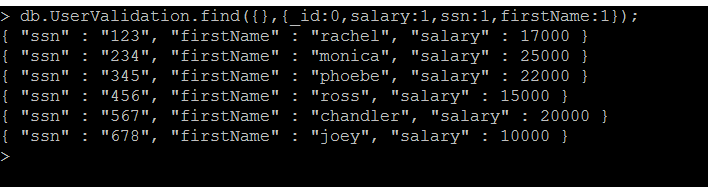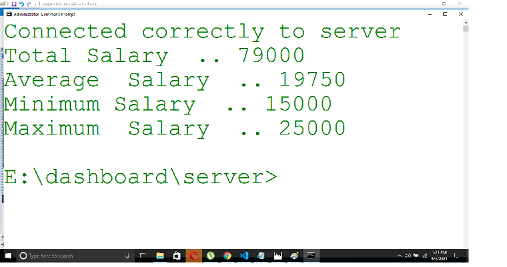Related Articles

# Aggregate Queries

• Last Updated : 16 Jul, 2021

MongoDB is a NoSQL document model database, and it is mainly used in Big Data analysis like election poll results, growth of an industry in the past few years, etc., For doing huge computations, in MongoDB, we use aggregation.

Aggregation groups the data from multiple documents and provides the summed up results, the average value from a large result set, min/max value from a large result set, etc. In this article, let us see how we can perform Aggregation using Mongoose.

Module Installation: Install the required module using the following command.

`npm install mongoose`

Database: Following is the sample data present in a collection in the MongoDB database.

```Database Name: UserDB
Collection Name: UserValidation```

The below image shows that the existence of the document in it. Specifically taken ssn and salary columns alone.

`db.UserValidation.find({},{_id:0,salary:1,ssn:1,firstName:1});`Existence of data in “UserValidation” collection

Project structure: It will look like this.Example 1: Summation of the salary values.

Syntax: As we are summing up the fields, we need a grouping operation(\$group) followed by summation (\$sum) as shown below.

```db.UserValidation.aggregate([
{
\$group: {
_id: null,
salarycount: {
\$sum: "\$salary"
}
}
}
]); ```

Filename: server.js

## Javascript

 `// Requiring module``const mongoose = require(``"mongoose"``);`` ` `// Assumption is in our local, we have "UserDB" ``// named mongoDB database available``mongoose.connect(``    ``"mongodb://localhost:27017/UserDB"``,``    ``function` `(err, db) {`` ` `        ``console.log(``"Connected correctly to server"``);`` ` `        ``// "UserValidation" named collection ``        ``// available and each document contains``        ``// "salary" named column``        ``var` `col = db.collection(``'UserValidation'``);`` ` `        ``// By applying aggregate functionality,``        ``// finding the summation of salary and ``        ``// the result is in "summedUpDocument"``        ``col.aggregate([{``            ``\$group:``                ``{ _id: ``null``, total: { \$sum: ``'\$salary'` `} }``        ``}]).toArray(``function` `(err, summedUpDocument) {`` ` `            ``// Summed up salary value can be printed``            ``// as like below``            ``console.log(summedUpDocument.total)``            ``db.close();``        ``})``    ``});`

Step to run the program: Run the server using the following command.

`node server.js`

Output:Example 2: Calculate location-based summation of salaries of employees.

Syntax: In order to provide additional filter conditions, we can use the \$match operator.

```db.UserValidation.aggregate([
{
\$match: {
\$eq: "Chennai"
}
}
},
{
\$group: {
_id: null,
salarycount: {
\$sum: "\$salary"
}
}
}]);  ```

Here we are matching the address column to have Chennai only. Hence, whichever documents matching with the value of the address to Chennai are only summed up.

Filename: server.js

## Javascript

 `// Requiring module``const mongoose = require(``"mongoose"``);`` ` `// Assumption is in our local, we have``// "UserDB" named mongoDB database``// available``mongoose.connect(``    ``"mongodb://localhost:27017/UserDB"``,``    ``function` `(err, db) {`` ` `        ``console.log(``"Connected correctly to server"``);`` ` `        ``// "UserValidation" named collection ``        ``// available and each document contains``        ``// "salary" named column``        ``var` `col = db.collection(``'UserValidation'``);`` ` `        ``// By applying aggregate functionality, ``        ``// finding the summation of salary ``        ``// Here applied match condition where ``        ``// matching address equal to ``        ``// Chennai and result will be in ``        ``// summedUpDocumentForChennai``        ``col.aggregate([``            ``{ \$match: { address: { \$eq: ``"Chennai"` `} } },``            ``{``                ``\$group:``                    ``{ _id: ``null``, total: { \$sum: ``'\$salary'` `} }``            ``}``        ``]).toArray(``function` `(err, summedUpDocumentForChennai) {`` ` `            ``// Summed up salary value can be printed``            ``// as like below``            ``console.log(summedUpDocumentForChennai.total)``            ``db.close();``        ``})``    ``});`

Step to run the program: Run the server using the following command.

`node server.js`

Output:Example 3: To get Average value/Minimum value/Maximum value

Syntax: We need to use avg functionality for average/min functionality for minimum/max functionality for maximum using the following syntax.

```db.UserValidation.aggregate([{
\$match: { address: { \$eq: "Chennai" } }
},
{
\$group: {
_id: null,
totalSalary: { \$sum: "\$salary" },
averageSalary: { \$avg: "\$salary" },
minimumSalary: { \$min: "\$salary" },
maximumSalary: { \$max: "\$salary" }
}
}
])```

Filename: server.js

## Javascript

 `// Requiring module``const mongoose = require(``"mongoose"``);`` ` `// Assumption is in our local, we have``// "UserDB" named mongoDB database ``// available``mongoose.connect(``    ``"mongodb://localhost:27017/UserDB"``, ``    ``function` `(err, db) {`` ` `    ``console.log(``"Connected correctly to server"``);`` ` `    ``// "UserValidation" named collection``    ``// available and each document contains``    ``// "salary" named column``    ``var` `col = db.collection(``'UserValidation'``);`` ` `    ``// By applying aggregate functionality,``    ``// finding the summation of salary ``    ``// Here applied match condition where``    ``// matching address equal to Chennai ``    ``// We are calculating total, average,``    ``// minimum and maximum amount``    ``col.aggregate([``        ``{ \$match: { address: { \$eq: ``"Chennai"` `} } },``        ``{``            ``\$group:``            ``{``                ``_id: ``null``, totalSalary: { \$sum: ``"\$salary"` `},``                ``averageSalary: { \$avg: ``"\$salary"` `},``                ``minimumSalary: { \$min: ``"\$salary"` `},``                ``maximumSalary: { \$max: ``"\$salary"` `}``            ``}``        ``}``    ``]).toArray(``function` `(err, projectFunctionality) {``        ``console.log(``"Total Salary  .."``, ``            ``projectFunctionality.totalSalary)`` ` `        ``console.log(``"Average  Salary  .."``, ``            ``projectFunctionality.averageSalary)`` ` `        ``console.log(``"Minimum Salary  .."``, ``            ``projectFunctionality.minimumSalary)`` ` `        ``console.log(``"Maximum  Salary  .."``, ``            ``projectFunctionality.maximumSalary)``        ``db.close();``    ``})``});`

Step to run the program: Run the server using the following command.

`node server.js`

Output:Note: By default, the _id field will be available for the output documents. We can use 1 to include a field in the output document and 0 to suppress.

Conclusion: Aggregate queries are very powerful and their functionality is similar to SQL in terms of group by clause(i.e. \$group) and having functionalities (i.e. \$match). MongoDB is a much powerful NoSQL documentum typed database and aggregate queries play a vital role in that.

References: https://docs.mongodb.com/manual/aggregation/

My Personal Notes arrow_drop_up